Solving Equilibrium Problems Involving Bases

Solving Equilibrium Problems Involving Bases

With minor modifications, the techniques applied to equilibrium calculations for acids are valid for solutions of bases in water.

Consider the calculation of the pH of an 0.10 M NH3 solution. We can start by writing an equation for the reaction between ammonia and water.

NH3(aq) + H2O(l)NH4+(aq) + OH-(aq)

Strict adherence to the rules for writing equilibrium constant expressions leads to the following equation for this reaction.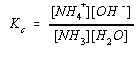But, taking a lesson from our experience with acid-dissociation equilibria, we can build the [H2O] term into the value of the equilibrium constant. Reactions between a base and water are therefore described in terms of a base-ionization equilibrium constant, Kb.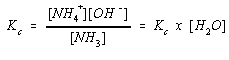For NH3, Kb is 1.8 x 10-5.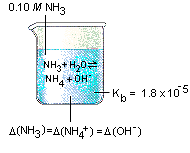We can organize what we know about this equilibrium with the format we used for equilibria involving acids.

 NH3(aq) + H2O(l)NH4+(aq) + OH-(aq) Kb = 1.8 x 10-5 Initial: 0.10 M 0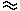0 Equilibrium: 0.10 -CCC

Substituting this information into the equilibrium constant expression gives the following equation.Kb for ammonia is small enough to allow us to consider the assumption thatC is small compared with the initial concentration of the base.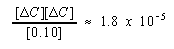Solving this approximate equation gives the following result.C1.3 x 10-3

This value ofis small enough compared with the initial concentration of NH3 to be ignored and yet large enough compared with the OH- ion concentration in water to ignore the dissociation of water. It can therefore be used to calculate the pOH of the solution.

pOH = - log (1.3 x 10-3) = 2.89

Which, in turn, can be used to calculate the pH of the solution.

pH = 14 - pOH = 11.11

Equilibrium problems involving bases are relatively easy to solve if the value of Kb for the base is known. The first step in many base equilibrium calculations involves determining the value of Kb for the reaction from the value of Ka for the conjugate acid.

As an example, let's calculate the pH of a 0.030 M solution of sodium benzoate (C6H5CO2Na) in water from the value of Ka for benzoic acid (C6H5CO2H): Ka = 6.3 x 10-5.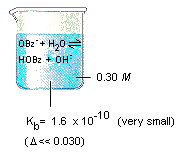Benzoic acid and sodium benzoate are members of a family of food additives whose ability to retard the rate at which food spoils has helped produce a 10-fold decrease in the incidence of stomach cancer. To save time and space, we'll abbreviate benzoic acid as HOBz and sodium benzoate as NaOBz. Benzoic acid, as its name implies, is an acid. Sodium benzoate is a salt of the conjugate base, the OBz- or benzoate ion.

Whenever sodium benzoate dissolves in water, it dissociates into its ions.

 H2O NaOBz(aq)Na+(aq) + OBz-(aq)

The benzoate ion then acts as a base toward water, picking up a proton to form the conjugate acid and a hydroxide ion.

OBz-(aq) + H2O(l)HOBz(aq) + OH-(aq)

The base-ionization equilibrium constant expression for this reaction is therefore written as follows.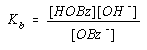The next step in solving the problem involves calculating the value of Kb for the OBz- ion from the value of Ka for HOBz.

The Ka and Kb expressions for benzoic acid and its conjugate base both contain the ratio of the equilibrium concentrations of the acid and its conjugate base. Ka is proportional to [OBz-] divided by [HOBz], and Kb is proportional to [HOBz] divided by [OBz-].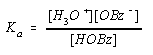Two changes have to made to derive the Kb expression from the Ka expression: We need to remove the [H3O+] term and introduce an [OH-] term. We can do this by multiplying the top and bottom of the Ka expression by the OH- ion concentration.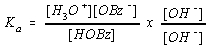Rearranging this equation gives the following result.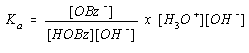The two terms on the right side of this equation should look familiar. The first is the inverse of the Kb expression, the second is the expression for Kw.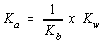Rearranging this equation gives the following result.

Ka x Kb = Kw

According to this equation, the value of Kb for the reaction between the benzoate ion and water can be calculated from Ka for benzoic acid.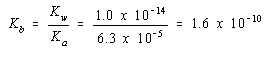Now that we know Kb for the benzoate ion, we can calculate the pH of an 0.030 M NaOBz solution with the techniques used to handle weak-acid equilibria. We start, once again, by building a representation for the problem.

 OBz-(aq) + H2O(l)HOBz(aq) + OH-(aq) Kb = 1.6 x 10-10 Initial: 0.030 M 00 Equilibrium: 0.030 -CCC

We then substitute this information into the Kb expression.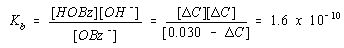Because Kb is relatively small, we assume thatC is small compared with 0.030.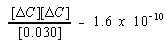We then solve the approximate equation for the value ofC.C2.2 x 10-6

The assumption thatC is small is obviously valid. We can therefore useC to calculate the pOH of the solution.

[OH-] =C2.2 x 10-6

pOH = - log [2.2 x 10-6] = 5.66

The problem asked for the pH of the solution, however, so we use the relationship between pH and pOH to calculate the pH.

pH = 14 - pOH = 8.34

Two assumptions were made in this calculation.

• We assumed thatC was small enough compared with the initial concentration of the base that it could be ignored in the [0.030 -C] term.
• We assumed that all of the OH- ion at equilibrium came from the reaction between the benzoate ion and water. (In other words, we ignored the contribution to the OH- ion concentration from the dissociation of water.)

We have already confirmed the validity of the first assumption. What about the second? The OH- ion concentration obtained from this calculation is 2.1 x 10-6 M, which is 21 times the OH- ion concentration in pure water. According to LeChatelier's principle, however, the addition of a base suppresses the dissociation of water. This means that the dissociation of water makes a contribution of significantly less than 5% to the total OH- ion concentration in this solution. It can therefore be legitimately ignored.

Two factors affect the OH- ion concentration in aqueous solutions of bases: Kb and Cb. We can ignore the dissociation of water when KbCb for a weak base is larger than 1.0 x 10-13. When KbCb is smaller than 1.0 x 10-13, we have to include the dissociation of water in our calculations.

 Practice Problem 5:Calculate the HOAc, OAc-, and OH- concentrations at equilibrium in an 0.10 M NaOAc solution. (HOAc: Ka = 1.8 x 10-5)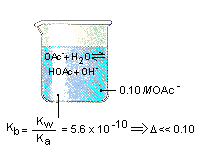Click here to check your answer to Practice Problem 5 Click here to see a solution to Practice Problem 5